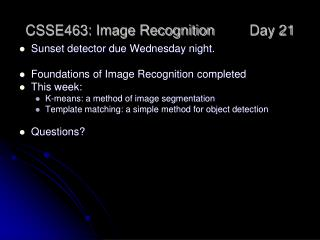DownloadDownload PresentationCSSE463: Image Recognition Day 21

# CSSE463: Image Recognition Day 21

Download Presentation## CSSE463: Image Recognition Day 21

- - - - - - - - - - - - - - - - - - - - - - - - - - - E N D - - - - - - - - - - - - - - - - - - - - - - - - - - -
##### Presentation Transcript

1. CSSE463: Image Recognition Day 21 • Sunset detector due Wednesday night. • Foundations of Image Recognition completed • This week: • K-means: a method of image segmentation • Template matching: a simple method for object detection • Questions?

2. An image to segment…

3. Segmentation • The process of breaking an image into regions. • Two types: • General-purpose • “One size fits all” • Very difficult… • Specialized • Intended for a specific domain (say fruit-, circle- or skin-finding) • Can be successful • One to right is created using the mean-shift algorithm • D. Comaniciu, P. Meer: Mean shift: A robust approach toward feature space analysis.IEEE Trans. Pattern Anal. Machine Intell, 24, 603-619, 2002. • EDISON code downloadable at http://www.caip.rutgers.edu/riul/research/robust.html

4. What properties can we use to segment? • Regions homogeneous wrt. color, texture, etc. • Simple interiors • Adjacent regions different (else merge) • Smooth boundaries

5. Approaches • Models • Uses an expected shape, color, etc. (fruit- and circle-finders) • Can use probabilities • Clustering • An unsupervised machine learning technique • No class labels used in learning! • Groups pixels “close” to each other by some metric. • Color distance, texture, intensity, spatial location, etc. • Regions are then found using connected components Q1

6. K-means clustering • Goal: given K clusters, assign each pixel to one of the clusters such that the total distance from each pixel to the center of its cluster is minimized. • We control C, the assignment of pixels to each cluster. (We will actually do this by specifying the location of their means) • D= total distance • K = # of clusters • x are pixels • Ck is the set of pixels in cluster k • mk is the center of cluster k • ||.|| is a distance Q2

7. K-means clustering Problems: • What’s K? • How do we know which pixel belongs to each cluster? • K-means is an answer to the second question. • K = # of clusters • x are pixels • Ck is the set of pixels in cluster k • mk is the center of cluster k • ||.|| is a distance

8. K-means clustering • Iterative process to group into k clusters. • Algorithm (Sonka, p 403; Forsyth&Ponce, p. 315; Shapiro, p. 282) • Initialize k cluster means • Repeat until convergence: • For each pixel, find the closest mean and assign it to that cluster • Re-compute the mean of all pixels assigned to the cluster • Label each pixel with its current cluster • Example on board using 2D spatial distance • Could you implement? Q3-5

9. K-means clustering • We are trying to find out where the clusters are and which points are assigned to each cluster. We iteratively solve half the problem. Notice the overall structure: • Repeat until convergence: • Assume you know where the cluster centers are. For each pixel, find the closest mean and assign it to that cluster • Assume you know which points belong to each cluster. Recompute the mean of all pixels assigned to the cluster • Label each pixel with its current cluster

10. K-means clustering • Pros: • Easy to implement • Finds local optimum • Global optimization (over all partitions) is infeasible • Cons: • The number of clusters, k, must be known in advance • Some clusters might have 0 points • Local optimum is only an approximation • Ideas: • Can re-run with several initializations • Can choose k based on observation or statistical means Q6

11. K-means clustering • K = # of clusters • x are pixels • Ck is the set of pixels in cluster k • mk is the center of cluster k • ||.|| is a distance: could be 2D distance in image or 3D Euclidean distance between colors (or combination of both) (On Lab: will produce disconnected regions) Q7-8

12. K-means results Original (120x160) K=3 K=5 K=7